# Solution assignment 10 The basics

### Assignment 10

Calculate without the aid of a calculator:.

### Solution

Use the fact that the value of a fraction does not change when numerator and denominator are divided by the same nonnegative number. We apply this rule and subsequently divide by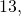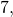and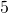, resulting in: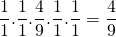As an example, the numerator contains(that is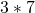) and the denominator contains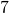(that is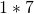). If we divide both numerator and denominator bythe result will be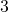in the numerator andin the denominator.